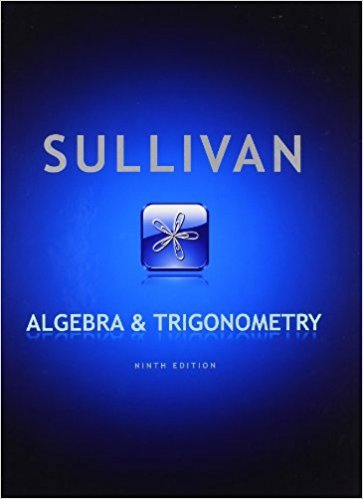×
×

# Solutions for Chapter 1.5: Algebra and Trigonometry 9th Edition## Full solutions for Algebra and Trigonometry | 9th Edition

ISBN: 9780321716569Solutions for Chapter 1.5

Solutions for Chapter 1.5
4 5 0 271 Reviews
18
4
##### ISBN: 9780321716569

Algebra and Trigonometry was written by and is associated to the ISBN: 9780321716569. Chapter 1.5 includes 125 full step-by-step solutions. This textbook survival guide was created for the textbook: Algebra and Trigonometry, edition: 9. This expansive textbook survival guide covers the following chapters and their solutions. Since 125 problems in chapter 1.5 have been answered, more than 57685 students have viewed full step-by-step solutions from this chapter.

Key Math Terms and definitions covered in this textbook
• Cholesky factorization

A = CTC = (L.J]))(L.J]))T for positive definite A.

• Condition number

cond(A) = c(A) = IIAIlIIA-III = amaxlamin. In Ax = b, the relative change Ilox III Ilx II is less than cond(A) times the relative change Ilob III lib II· Condition numbers measure the sensitivity of the output to change in the input.

• Covariance matrix:E.

When random variables Xi have mean = average value = 0, their covariances "'£ ij are the averages of XiX j. With means Xi, the matrix :E = mean of (x - x) (x - x) T is positive (semi)definite; :E is diagonal if the Xi are independent.

• Elimination matrix = Elementary matrix Eij.

The identity matrix with an extra -eij in the i, j entry (i #- j). Then Eij A subtracts eij times row j of A from row i.

• Free columns of A.

Columns without pivots; these are combinations of earlier columns.

• Full column rank r = n.

Independent columns, N(A) = {O}, no free variables.

• Full row rank r = m.

Independent rows, at least one solution to Ax = b, column space is all of Rm. Full rank means full column rank or full row rank.

• Gauss-Jordan method.

Invert A by row operations on [A I] to reach [I A-I].

• Hermitian matrix A H = AT = A.

Complex analog a j i = aU of a symmetric matrix.

• Length II x II.

Square root of x T x (Pythagoras in n dimensions).

• Lucas numbers

Ln = 2,J, 3, 4, ... satisfy Ln = L n- l +Ln- 2 = A1 +A~, with AI, A2 = (1 ± -/5)/2 from the Fibonacci matrix U~]' Compare Lo = 2 with Fo = O.

• Orthogonal matrix Q.

Square matrix with orthonormal columns, so QT = Q-l. Preserves length and angles, IIQxll = IIxll and (QX)T(Qy) = xTy. AlllAI = 1, with orthogonal eigenvectors. Examples: Rotation, reflection, permutation.

• Orthogonal subspaces.

Every v in V is orthogonal to every w in W.

• Orthonormal vectors q 1 , ... , q n·

Dot products are q T q j = 0 if i =1= j and q T q i = 1. The matrix Q with these orthonormal columns has Q T Q = I. If m = n then Q T = Q -1 and q 1 ' ... , q n is an orthonormal basis for Rn : every v = L (v T q j )q j •

• Rank one matrix A = uvT f=. O.

Column and row spaces = lines cu and cv.

• Rank r (A)

= number of pivots = dimension of column space = dimension of row space.

• Row picture of Ax = b.

Each equation gives a plane in Rn; the planes intersect at x.

• Spectrum of A = the set of eigenvalues {A I, ... , An}.

Spectral radius = max of IAi I.

• Symmetric factorizations A = LDLT and A = QAQT.

Signs in A = signs in D.

• Volume of box.

The rows (or the columns) of A generate a box with volume I det(A) I.

×